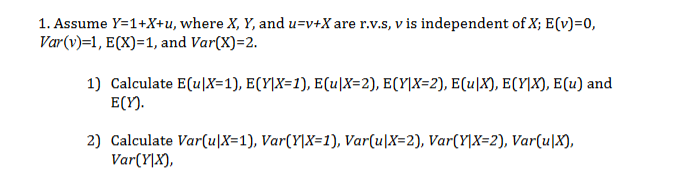# 1. Assume Y=1+X+u, where X, Y, and u=v+Xare r.v.S, v is independent ofX; E(v)=0,Var(v)=1, ECX) 1, and Var(X) 21) Calculate E(uX=1), E(Y]X=1), E(u|X=2), E(Y]X=2}, E(uX), ECY]X), E(u) andE(Y)2) Calculate Var(u|X=1), Var(Y\X=1), Var(u|X=2), Var(Y]X=2), Var(u|X),VarYX),

Question
444 views

Part 1 and 2help_outlineImage Transcriptionclose1. Assume Y=1+X+u, where X, Y, and u=v+Xare r.v.S, v is independent ofX; E(v)=0, Var(v)=1, ECX) 1, and Var(X) 2 1) Calculate E(uX=1), E(Y]X=1), E(u|X=2), E(Y]X=2}, E(uX), ECY]X), E(u) and E(Y) 2) Calculate Var(u|X=1), Var(Y\X=1), Var(u|X=2), Var(Y]X=2), Var(u|X), VarYX), fullscreen
check_circle

Step 1

1)

Given Data

Y= 1+X+u

u = v + X

E(v) = 0

Var(v) = 1

E(X) =1

Var(x) =2

1)

E(u|X = 1)

E(u|X = 1) = E(v+X| X=1) = E(v|X =1) + E(X|X =1) =  0 +1 = 1

E(v|X =1) = 0 because v is independent of x

E(y|X = 1) = E(1+X+u|X =1) = E(1+X+ v+X|X=1) = E(1+2X+v|X =1) = 1+2X + 0

=1+2*1= 3

E(v|X =1) = 0 because v is independent of x

E(u|X = 2) = E(v+X| X=2) = E(v|X =2) + E(X|X =2) =  0 +2 = 2

E(Y|X = 2) = E(1+X+u|X =2) = E(1+X+ v+X|X=2) = E(1+2X+v|X =2) =1+2X + 0

= 1+2*2 + 0 = 5

E(u|X ) = E(v+X|X) = E(v|X ) + E(X|X ) =...

### Want to see the full answer?

See Solution

#### Want to see this answer and more?

Solutions are written by subject experts who are available 24/7. Questions are typically answered within 1 hour.*

See Solution
*Response times may vary by subject and question.
Tagged in

### Statistics